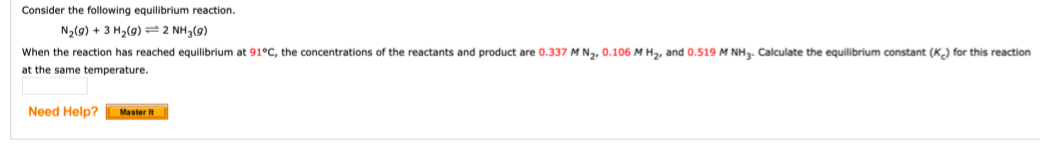# Consider the following equilibrium reaction. N2(g) + 3 H2(g) -- 2 NH3(g) When the reaction has reached equilibrium at 91°C, the concentrations of the reactants and product are 0.337 M N2, 0.106 M H2, and 0.519 M NH3. Calculate the equilibrium constant (Kg) for this reaction at the same temperature